# The Excel Sum Function

You saw a simple way to add up in the previous section. Enter an equals sign, followed by the cells you want Excel to add up:

= B4 + B5 + B6 + B7

But this is not a good way to add up in Excel: it could get very tedious indeed if you had to type out say 50 cell references by hand. The easy way is to get Excel to do the work for you. That's where SUM comes in.

## The Excel SUM function

The SUM function is used to add things up, and saves you the bother of typing out lots of cell names and numbers. It looks like this:

=SUM( )

In between the round brackets, you type what you want Excel to add up. Look at our spreadsheet again. Here it is in Excel 2007:In Excel 2010 and 2016 you'll have this less colourful version:We want to add up the numbers under the Monday heading, and place the answer in cell B9.

So with cell B9 selected again, click into your formula bar. If you're following along from the previous lesson, you should have this in cell B9:If you have an equals sign before B4, delete it and press the enter key. Now position your cursor at the start of the line, before the "B" of B4.

Type an equals sign first, then the letter SU of SUM.

As soon as you start typing, Excel will present you with a drop down list of available functions. Click once with the left mouse button on SUM to highlight it: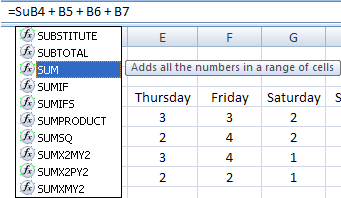Now double click on SUM. Excel will add the "M" for you, and the left bracket. It will also highlight the cells in your formula: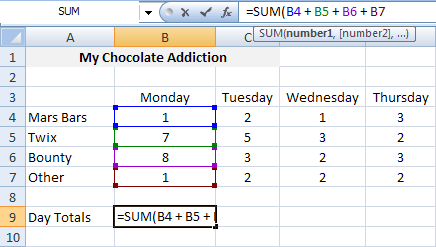Now press the Enter key on your keyboard. Excel will add the right bracket, and work out the SUM for you:Now click back on cell B9, and look at the Name box (just above the A column, in our image). It has B9 in it. The formula bar to the right shows you which formula you have in the active cell (B9).

Another way to use the SUM function is this:

• Click into cell C9. Type =SUM.
• Double click the SUM function from the dropdown list, as before. Now click into cell C4:
•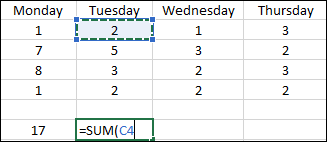• Keep your left mouse button held down and drag to cell C7. Excel will highlight these cells and put the cell references into the formula for you:
•• Type and end round bracket, and then press the enter key on your keyboard.

If you wanted to, you could hold down the CTRL key on your keyboard and select different cells on your spreadsheet. These would then get added between the round brackets of SUM. In other words, if you want to include cells that are not in the same column, or are not next to each other, then you can.

An easier way to add up number with the SUM function is to use a colon (:) The colon is a shorthand way of adding up consecutive cells. Instead of typing out all those cell references like this:

=SUM(B4 + B5 + B6 + B7)

You can just type out the first cell reference, then a colon, then the last cell reference. Like this:

=Sum(B4: B7)

Excel will then add up the numbers in cells B4 to B7. It knows what the colon means!

• Click into cell B9, if it's not already active
• Now click on the cell with your right mouse button
• You'll see a menu appear:• From the menu, select Clear Contents by clicking the item with your left mouse button
• This will clear the formula from the formula bar
• Now click back inside of the formula bar and type the following:

=Sum(B4:B7)When you have the formula typed out, hit the Enter key on your keyboard. Excel will add up the numbers for you, and place the correct answer in cell B9.

If everything went well, you should have an answer of 17 in cell B9. Fortunately, we can use AutoFill for the rest of the answers.

• Place your mouse pointer to the bottom right of cell B9
• The pointer will turn into a thin black cross: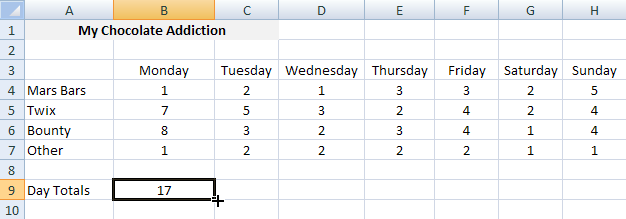• Hold down your left mouse button
• Keep it held down, and drag your mouse to cell H9: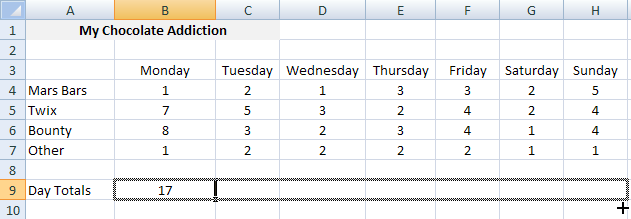With your mouse pointer over cell H9, let go of the left button. Excel will AutoFill the rest of the formulas. It uses the same formula from cell B9 to get the answers, and just alters all the cell references. Without AutoFill, you'd have to type it all out yourself!

The answers on Row 9 of your spreadsheet should be the same as ours in the image below:Notice the formula bar in the image. It shows the formula in cell H9. This is:

=Sum(H4:H7)

The formula we started with was:

=Sum(B4:B7)

Excel has changed the letters for us, but not the numbers. In other words, it's adding up the columns.

If you think of the colon as the word TO, it should make sense:

Add up the cells B4 TO B7
Add up the cells H4 TO H7

In the next section, you'll get some more practice with this spreadsheet, and with the SUM Function. So don't forget to save the work you've done so far!

<--Back to the Excel Contents Page